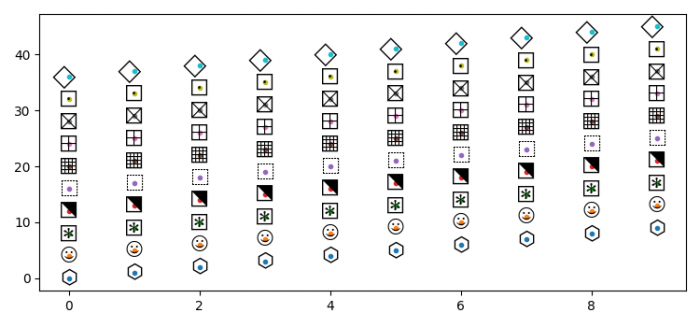# How to use Font Awesome symbol as marker in matplotlib?

To use Font Awesome symbol as a marker, we can take the following steps −

• Set the figure size and adjust the padding between and around the subplots.
• Create a list of symbols; has to be plotted.
• Create x and y data points using numpy.
• Create a new figure or activate an existing figure using figure() method.
• Iterate the symbols and use it while plotting a line.
• To display the figure, use show() method.

## Example

import numpy as np
import matplotlib.pyplot as plt

plt.rcParams["figure.figsize"] = [7.50, 3.50]
plt.rcParams["figure.autolayout"] = True

symbols = [u'\u2B21', u'\u263A', u'\u29C6', u'\u2B14', u'\u2B1A', u'\u25A6', u'\u229E', u'\u22A0', u'\u22A1', u'\u20DF']

x = np.arange(10)
y = np.arange(10)

plt.figure()

for i, symbol in enumerate(symbols):
y2 = y + 4*i
plt.plot(x, y2, '.')
for x0, y0 in zip(x, y2):
plt.text(x0, y0, symbol, fontname='STIXGeneral', size=15, va='center', ha='center', clip_on=True)

plt.show()

## Output